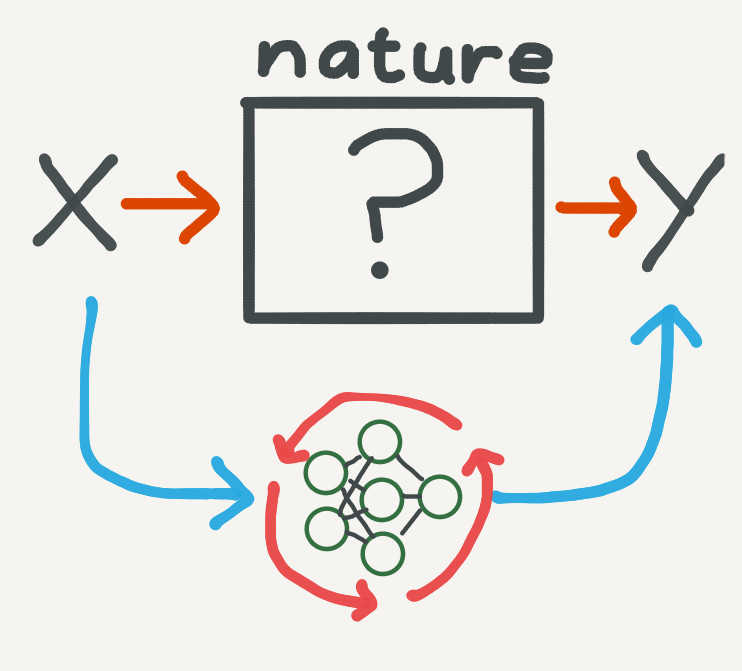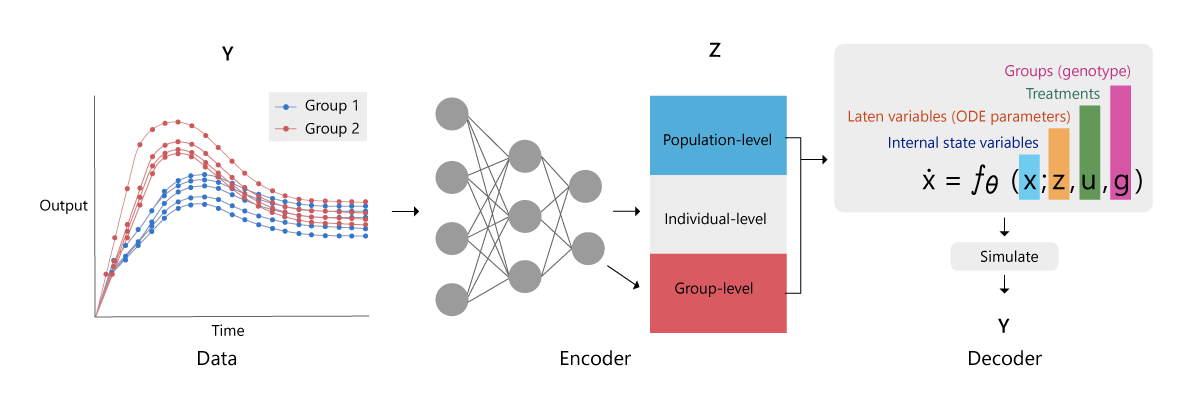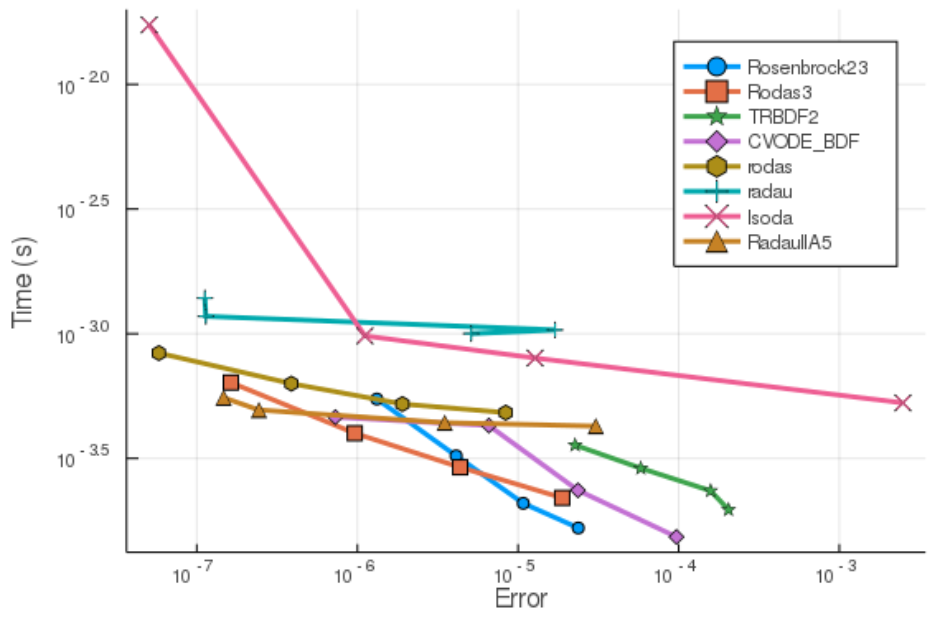# Differential equation, Logistic function, Equation, Mathematics, Neural network, Partial differential equation

On Oct 31, 2020
RT @PyTorchLightnin: Blazing fast PDE solver from @Caltech and @AnimaAnandkumar https://t.co/mWPrRn16yo
Open

### AI has cracked a key mathematical puzzle for understanding our worldUnless you’re a physicist or an engineer, there really isn’t much reason for you to know about partial differential equations. I know. After years of poring over them in undergrad while ...

### AI Has Cracked a Key Mathematical Puzzle For Understanding Our WorldAn anonymous reader shares a report: Unless you're a physicist or an engineer, there really isn't much reason for you to know about partial differential equations. I know. After years of ...

### Physics Informed Deep LearningData-driven solutions and discovery of Nonlinear Partial Differential Equations

### Neural Ordinary Differential EquationsNeural ordinary differential equations Chen et al., NeurIPS’18 ‘Neural Ordinary Differential Equations’ won a best paper award at NeurIPS last month. It’s not an easy piece (at least not ...

### Understanding Neural ODE'sIn this blogpost I explore how ODE’s can be used to solve data modelling problems. I take a deep dive into the data modelling problem at hand and present ODE’s (which model rates of change) ...

### Neural networks as Ordinary Differential EquationsRecently I found a paper being presented at NeurIPS this year, entitled Neural Ordinary Differential Equations, written by Ricky Chen, Yulia Rubanova, Jesse Bettencourt, and David Duvenaud ...

### Workshop AbstractThese two methods are not only closely related to each other but also offer complementary strengths: the modelling power and interpretability of differential equations, and the ...

### Efficient inference for dynamical models using variational autoencodersResearchers at Microsoft Research Cambridge have released Python code and data for their work using machine learning to efficiently parameterize prescribed and neural dynamical systems ...

### SciML: An Open Source Software Organization for Scientific Machine LearningComputational scientific discovery is at an interesting juncture. While we have mechanistic models of lots of different scientific phenomena, and reams of data being generated from ...Other neural nets haven’t progressed beyond simple addition and multiplication, but this one calculates integrals and solves differential equations.

### Deep Learning on Bad Time Series Data: Corrupt, Sparse, Irregular and UglyHow do you train neural networks on time series that are non-uniformly sampled,  irregularly sampled, have non-equidistant timesteps, or have missing or corrupt values? In the following ...

### Supercomputing Simulations and Machine Learning Help Improve Power PlantsHigh-performance computing resources and data-driven machine learning helped University of Stuttgart researchers model how coal, nuclear, and geothermal power plants could be retrofitted ...